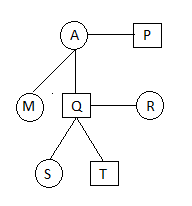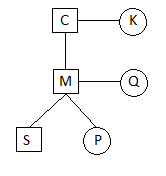New Student User - Use Code HELLO

# Blood Relation Quiz For IBPS PO: Part 2

Direction(Q1-3): Q is the son of A. P is the husband of A. Q has two children S and T. S is the daughter of R. M is the sister of Q. T is the brother of S.

Q.1 How is M related to R?
A. Sister
B. Mother
C. Sister in law
D. Aunt
E. None of these

Q.2 How many females in the family?
A. 4
B. 5
C. 3
D. 2
E. None of these

Q.3 How many males in the family?
A. 4
B. 3
C. 2
D. 5
E. None of these

Direction(Q4-5): In a family there are two couples, two son and one daughter.
• C is the grandfather of S.
• S is the son of M.
• K is the grandmother of P and is the wife of C.
• P is the daughter of Q.

Q.4 How is P related to K?
A. Grandson
B. Son
C. Granddaughter
D. Daughter
E. None of these

Q.5 Which of the following is a pair of couple?
A. MQ
B. CP
C. SP
D. KQ
E. None of these

#### 1. Ans. C.

Solution:#### 2. Ans. A.

Solution:#### 3. Ans. B.

Solution:#### 4.Ans. C.

Solution:#### 5. Ans. A.

Solution: